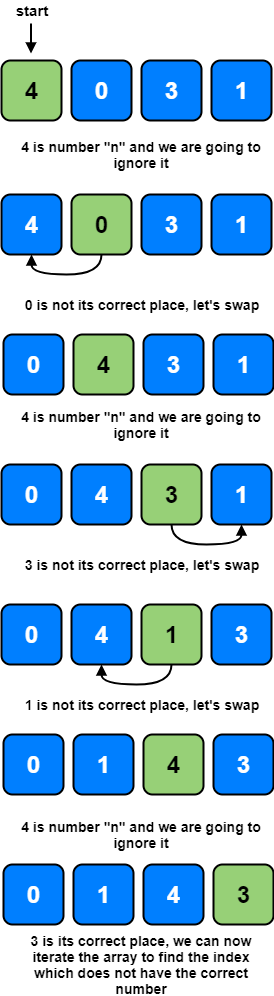# Coding Patterns: Cyclic Sort

In Coding Patterns series, we will try to recognize common patterns underlying behind each algorithm question, using real examples from Leetcode.

Previous posts were about Sliding Window, Two Pointers, Fast & Slow Pointers and Merge Intervals patterns and today, we will introduce Cyclic Sort pattern which is very useful to solve the problems involving arrays containing numbers in a given range, finding the missing or duplicate numbers.

## Problem: Missing Number

LeetCode 268 - Missing Number [easy]

Given an array containing n distinct numbers taken from 0, 1, 2, …, n, find the one that is missing from the array.

Example 1:

``````Input: [3, 0, 1]
Output: 2
``````

Example 2:

``````Input: [9, 6, 4, 2, 3, 5, 7, 0, 1]
Output: 8
``````

Note:

Your algorithm should run in linear runtime complexity. Could you implement it using only constant extra space complexity?

### Cyclic Sort Solution

As we know, the input array contains numbers in the range of `0` to `n`. We can use this fact to devise an efficient way to sort the numbers.

Since all numbers are unique, we can try placing each number at its correct place, for example, placing 0 at index `0`, placing 1 at index `1`, and so on.

Once we have every number in its correct place, we can iterate the array to find the index which does not have the correct number, and that index will be our missing number.

Since the array will have `n` numbers, which means array indices will range from `0` to `n-1`. Therefore, we will ignore the number `n` as we can’t place it in the array, so => `nums[i] < len(nums)```````class Solution:
def missingNumber(self, nums: List[int]) -> int:
start = 0

while start < len(nums):
num = nums[start]
if num < len(nums) and num != start:
nums[start], nums[num] = nums[num], nums[start]
else:
start += 1

for i in range(len(nums)):
if nums[i] != i:
return i

return len(nums)
``````

Time Complexity: O(N) + O(N - 1) which is asymptotically equivalent to O(N)

Space Complexity: O(1), algorithm runs in constant space.

## How to identify?

This approach is quite useful when dealing with numbers in a given range and asking to find the duplicates/missing ones etc.

When the problem involving arrays containing numbers in a given range, you should think about Cyclic Sort pattern.

Tags:

Categories:

Updated: